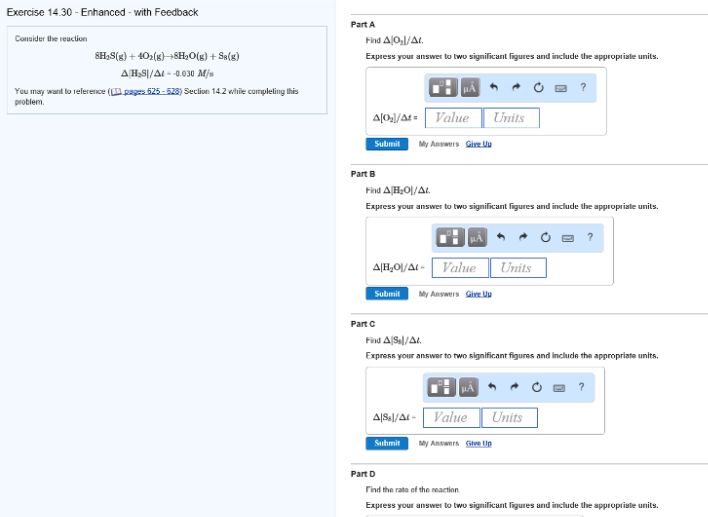# Problem: Consider the reaction 8H2S(g) + 4O2(g) → 8H2O(g) + S8(g) Δ[H2S]/Δt = -0.030 M/s A. Find Δ[O2]/Δt. B. Find Δ[H2O]/Δt. C. Find Δ[S8]/Δt.D. Find the rate of the reaction.

🤓 Based on our data, we think this question is relevant for Professor Roychowdhury's class at RUTGERS.

###### FREE Expert Solution###### Problem Details

Consider the reaction

8H2S(g) + 4O2(g) → 8H2O(g) + S8(g)

Δ[H2S]/Δt = -0.030 M/s

A. Find Δ[O2]/Δt.

B. Find Δ[H2O]/Δt.

C. Find Δ[S8]/Δt.

D. Find the rate of the reaction.